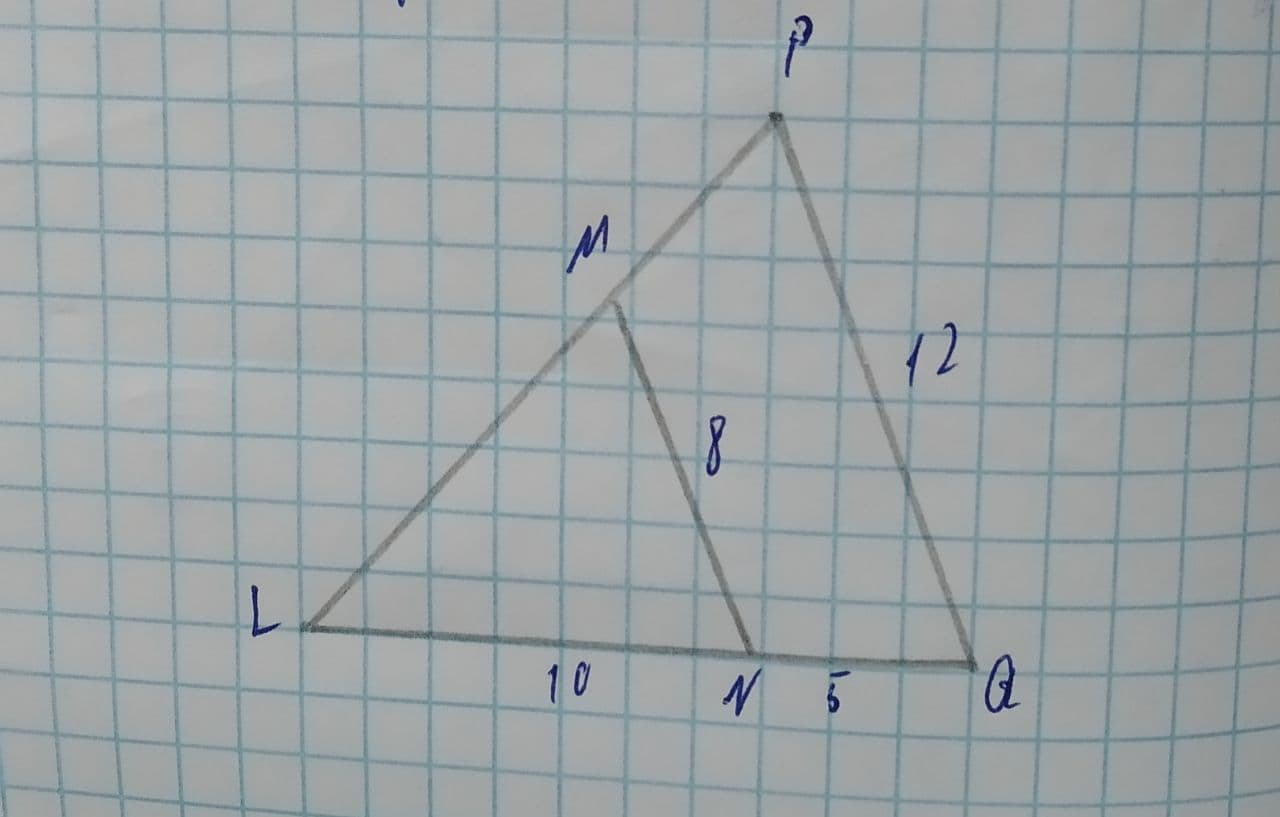# Determine if the triangles are similar \triangle LNM\sim \triangleDaniaal Sanchez 2021-08-09 Answered
Determine if the triangles are similar.
If they are, complete the similarity statement and indicate the theorem that proves similarity.
If they are not similar, select "not similar" for both entries.$\mathrm{△}LNM\sim \mathrm{△}$? by ?
You can still ask an expert for help

• Questions are typically answered in as fast as 30 minutes

Solve your problem for the price of one coffee

• Math expert for every subject
• Pay only if we can solve itwornoutwomanC
Step 1 Given
The triangle $\mathrm{△}LNM$ & $\mathrm{△}LQP$ and the sides are given to us is
MN=8
PQ=12
LN=10
NQ=5
Step 2
First, we will take two triangles $\mathrm{△}LNM$ & $\mathrm{△}LQP$ and seeing
$\frac{MN}{PQ}=\frac{8}{12}=\frac{2}{3}$
$\frac{LN}{LQ}=\frac{LN}{LN+NQ}=\frac{10}{10+15}=\frac{10}{15}=\frac{2}{3}$
We can see that the ratio are equal so,
$\frac{MN}{PQ}=\frac{LN}{LQ}=\frac{2}{3}$
And we can see that $\mathrm{\angle }L$ is common in both triangles
Step 3
Therefore, we can see that 2 sides and 1 angle is used in the similarity of the triangles.
Hence, we can say that by SAS similarity is used to show 2 triangles similar.
so,
$\mathrm{△}LNM\sim \mathrm{△}LQP$